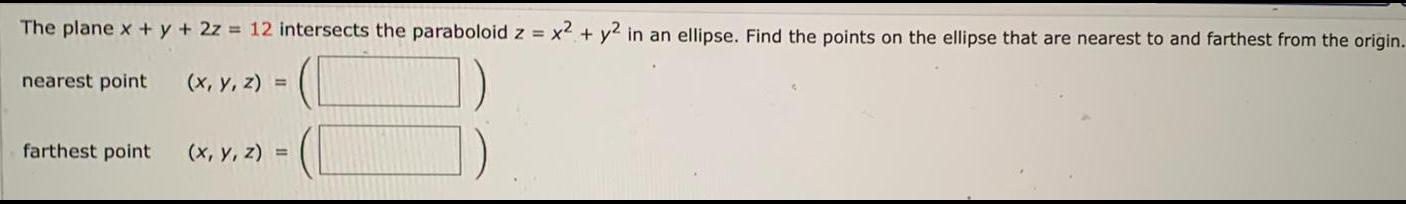Question:

# The plane x + y + 2z = 12 intersects the paraboloid z = x²

Last updated: 7/16/2022The plane x + y + 2z = 12 intersects the paraboloid z = x² + y² in an ellipse. Find the points on the ellipse that are nearest to and farthest from the origin. nearest point (x, y, z) = farthest point (x, y, z) =